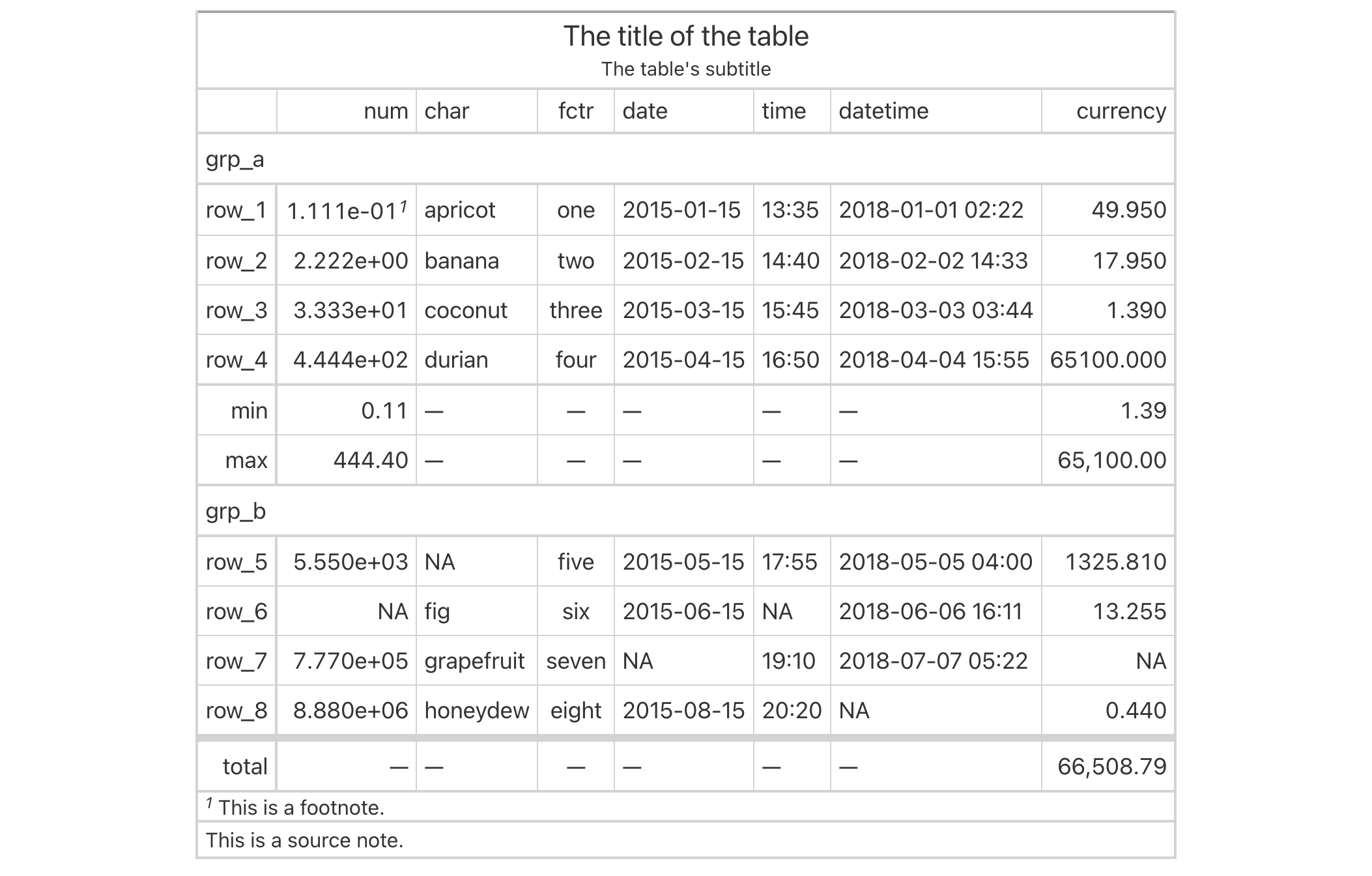# opt_table_lines: Option to set table lines to different extents In gt: Easily Create Presentation-Ready Display Tables

 opt_table_lines R Documentation

## Option to set table lines to different extents

### Description

The `opt_table_lines()` function sets table lines in one of three possible ways: (1) all possible table lines drawn (`"all"`), (2) no table lines at all (`"none"`), and (3) resetting to the default line styles (`"default"`). This is great if you want to start off with lots of lines and subtract just a few of them with `tab_options()` or `tab_style()`. Or, use it to start with a completely lineless table, adding individual lines as needed.

### Usage

```opt_table_lines(data, extent = c("all", "none", "default"))
```

### Arguments

 `data` A table object that is created using the `gt()` function. `extent` The extent to which lines will be visible in the table. Options are `"all"`, `"none"`, or `"default"`.

### Value

An object of class `gt_tbl`.

### Examples

Use `exibble` to create a gt table with a number of table parts added. Then, use the `opt_table_lines()` function to haves lines everywhere there can possibly be lines.

```exibble %>%
gt(rowname_col = "row", groupname_col = "group") %>%
summary_rows(
groups = "grp_a",
columns = c(num, currency),
fns = list(
min = ~min(., na.rm = TRUE),
max = ~max(., na.rm = TRUE)
)) %>%
grand_summary_rows(
columns = currency,
fns = list(
total = ~sum(., na.rm = TRUE)
)) %>%
tab_source_note(source_note = "This is a source note.") %>%
tab_footnote(
footnote = "This is a footnote.",
locations = cells_body(columns = 1, rows = 1)
) %>%
title = "The title of the table",
subtitle = "The table's subtitle"
) %>%
opt_table_lines()
```### Function ID

9-7

Other table option functions: `opt_align_table_header()`, `opt_all_caps()`, `opt_css()`, `opt_footnote_marks()`, `opt_horizontal_padding()`, `opt_row_striping()`, `opt_stylize()`, `opt_table_font()`, `opt_table_outline()`, `opt_vertical_padding()`